# Six times

6 times a number has 2 subtracted from it. The result it thrilled . the answer is the same if you add 6 to twice the number and multiply the result by 2

x =  7

### Step-by-step explanation:Did you find an error or inaccuracy? Feel free to write us. Thank you!Tips to related online calculators
Do you have a linear equation or system of equations and looking for its solution? Or do you have a quadratic equation?

## Related math problems and questions:

• Unknown numberSamuel wrote unknown number. Then he had add 200000 to the number and the result multiply by three. When it calculated he was surprised, because the result would have received anyway, if write digit to the end of original number. Find unknown number.
• NumberI think the number. If I add to its third seven I get same as when to its quarter add 8. Which is the number?
• I thinkI think a number. When I multiply it by five, and after that I subtract 477, I get the same number as if I multiplied it twice. What number do I think?
• Division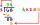Divide by the number 0.2 is the same as multiply by what number?
• Division by zero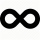Fraction 5 by 2. if 3 is added to numerator and 2 is subtracted from the denominator then the new fraction is:
• Evaluate 11Multiply the quotient of 6 and 2 by 3, then add 1. Add 1 and 6, then divide by 2 and multiply by 3. Divide 6 by the product of 2 and 3, then add 1.
• Unknown numberI think the number. I'll reduce it to its one-third. The result is then increased by one-third, and I get the number 12.
• Four paversFour pavers would pave the square in 18 days. How many pavers do you need to add to done work in 12 days?
• Mysterious number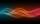The magician thinks the number: "The mysterious number is first divided by minus five, dividing the result by three, multiplying the number by ten, and dividing the resulting number by minus four. This gives result 5. Can you reveal the mysterious number?
• Unknown number 5I think of an unknown number. If we enlarge it five times then subtract 3 and the result decreases by 75% we get one greater than the number. What number am I thinking of?
• Two-digit numberI am a two-digit number less than 20. When I divided by three, then you get the rest 1 and when you divide me by four you get also rest 1. What number am I?
• By sixFrom the digits 1,2,3,4 we create the long integer number 123412341234. .. .. , which will have 962 digits. Is this number divisible by 6?
• Factors 2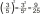A number has 3 identical factors. If 1 of them is 18. Find the number step by step
• Examination 2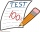In an examination, a teacher awards 2 marks for every correct answer and subtract a mark for every wrong answer. If there are 20 questions and a child scored 25 marks how many correct answer did the child get?
• Evaluate valueEvaluate value of the following expressions: 1. The product of nine and a number 12, decreased by six 2. Eight decreased by three times a number 2. 3. The quotient of twice a number 49 and seven.
• Unknown xIf we add to unknown number 21, then divide by 6 and then subtract 51, we get back an unknown number. What is this unknown number?
• Equation with mixed 2A number, X, is subtracted from 8 1/4. The result is 12 3/5. What is the value of X?